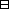## Group Theory and Linear Algebra

Last updated: 17 September 2014

## Lecture 4: Functions

Functions are for comparing sets.

Let $S$ and $T$ be sets. A function from $S$ to $T$ is a subset $f$ of $S×T,$ $f={(s,f(s)) | s∈S},$ such that

 (a) If $s\in S$ then there exists $t\in T$ such that $\left(s,t\right)\in f\text{.}$ (b) If $s\in S,$ and ${t}_{1},{t}_{2}\in T$ and $\left(s,{t}_{1}\right),\left(s,{t}_{2}\right)\in f$ then ${t}_{1}={t}_{2}\text{.}$
The function $f$ is an assignment assigning a mark $f\left(s\right)$ from $T$ to each $s\in S\text{.}$ Write $f:S⟶T s⟼f(s) or S⟶fT.$

A function $f:S\to T$ is injective if it satisfies: if ${s}_{1},{s}_{2}\in S$ and $f\left({s}_{1}\right)=f\left({s}_{2}\right)$ then ${s}_{1}={s}_{2}\text{.}$

A function $f:S\to T$ is surjective if it satisfies: if $t\in T$ then there exists $s\in S$ such that $f\left(s\right)=t\text{.}$

A function $f:S\to T$ is bijective if it is injective and surjective.

Let $f:S\to T$ and $g:S\to T$ be funtions. The functions $f$ and $g$ are equal if they satisfy if $s\in S$ then $f\left(s\right)=g\left(s\right)\text{.}$

Let $f:S\to T$ and $g:T\to U$ be functions. The composition of $g$ and $f$ is the function $g∘f:S⟶Tgiven by (g∘f)(s)=g (f(s)).$

Let $S$ be a set. The identity function on $S$ is the function $idS:S⟶Sgiven by idS(s)=s.$

Let $f:S\to T$ be a function. An inverse function to $f:S\to T$ is a function $g:T\to S$ such that $g∘f=idSand f∘g=idT.$

Let $f:S\to T$ be a function.

 (a) An inverse function to $f$ exists if and only if $f$ is bijective. (b) If an inverse function to $f$ exists then it is unique.Proof.

Assume $f:S\to T$ is a function.

(a)
To show: An inverse function to $f$ exists if and only if $f$ is bijective.
$⇒$ Assume that an inverse function to $f$ exists: $g:T\to S$ such that $g\circ f={\text{id}}_{S}$ and $f\circ g={\text{id}}_{T}\text{.}$
To show: $f$ is bijective.
To show:
 (1) $f$ is injective. (2) $f$ is surjective.
(1)
 To show: If ${s}_{1},{s}_{2}\in S$ and $f\left({s}_{1}\right)=f\left({s}_{2}\right)$ then ${s}_{1}={s}_{2}\text{.}$ Assume ${s}_{1},{s}_{2}\in S$ and $f\left({s}_{1}\right)=f\left({s}_{2}\right)\text{.}$ To show: ${s}_{1}={s}_{2}\text{.}$ $s1 = idS(s1)= (g∘f)(s1) =g(f(s1)) = g(f(s2))= (g∘f)(s2) =idS(s2)=s2.$
(2)
 To show: If $t\in T$ then there exists $s\in S$ such that $f\left(s\right)=t\text{.}$ Assume $t\in T\text{.}$ To show: There exists $s\in S$ such that $f\left(s\right)=t\text{.}$ Let $s=g\left(t\right)\text{.}$ To show: $f\left(s\right)=t\text{.}$ $f(s)= f(g(t))= (f∘g)(t)= idT(t)=t.$
So $f$ is bijective.
$⇐$
To show: If $f:S\to T$ is bijective then an inverse function $g:T\to S$ exists.
Assume $f:S\to T$ is bijective.
To show: There exists $g:T\to S$ such that $g∘f=idSand f∘g=idT.$
Let $g:T\to S$ be given by $g={(t,s)∈T×S | f(s)=t}.$
To show:
 (a) $g$ is a function. (b) $g\circ f={\text{id}}_{S}\text{.}$ (c) $f\circ g={\text{id}}_{T}\text{.}$
(a)
To show:
 (aa) If $t\in T$ then there exists $s\in S$ such that $\left(t,s\right)\in g\text{.}$ (ab) If $t\in T$ and ${s}_{1},{s}_{2}\in S$ and $\left(t,{s}_{1}\right)\in g$ and $\left(t,{s}_{2}\right)\in g$ then ${s}_{1}={s}_{2}\text{.}$
(aa)
 Assume $t\in T\text{.}$ To show: There exists $s\in S$ such that $\left(t,s\right)\in g\text{.}$ To show: There exists $s\in S$ such that $f\left(s\right)=t\text{.}$ This holds since $f$ is surjective.
(ab)
 Assume $t\in T,$ ${s}_{1},{s}_{2}\in S$ and $\left(t,{s}_{1}\right),\left(t,{s}_{2}\right)\in g\text{.}$ To show: ${s}_{1}={s}_{2}\text{.}$ Since $\left(t,{s}_{1}\right),\left(t,{s}_{2}\right)\in g,$ then $f\left({s}_{1}\right)=t$ and $f\left({s}_{2}\right)=t\text{.}$ Since $f$ is injective, ${s}_{1}={s}_{2}\text{.}$
(b)
 To show: $g\circ f={\text{id}}_{S}\text{.}$ To show: If $s\in S$ then $\left(g\circ f\right)\left(s\right)={\text{id}}_{S}\left(s\right)\text{.}$ Assume $s\in S\text{.}$ To show: $\left(g\circ f\right)\left(s\right)={\text{id}}_{S}\left(s\right)\text{.}$ $(g∘f)(s)=g(f(s))=s1,$ where ${s}_{1}\in S$ such that $f\left({s}_{1}\right)=f\left(s\right)\text{.}$ Since $f$ is injective, ${s}_{1}=s\text{.}$ So $\left(g\circ f\right)\left(s\right)=s={\text{id}}_{S}\left(s\right)\text{.}$
(c)
 To show: $\left(f\circ g\right)={\text{id}}_{T}\text{.}$ To show: If $t\in T$ then $\left(f\circ g\right)\left(t\right)={\text{id}}_{T}\left(t\right)\text{.}$ Assume $t\in T\text{.}$ To show: $\left(f\circ g\right)\left(t\right)={\text{id}}_{T}\left(t\right)\text{.}$ $(f∘g)(t)=f(g(t))=f(s),$ where $s\in S$ such that $f\left(s\right)=t\text{.}$ So $\left(f\circ g\right)\left(t\right)=f\left(s\right)=t={\text{id}}_{T}\left(t\right)\text{.}$
So $g$ is an inverse function to $f\text{.}$
(b)
 To show: The inverse function to $f:S\to T$ is unique. Assume ${g}_{1}:T\to S$ and ${g}_{2}:T\to S$ are inverse functions to $f\text{.}$ To show: ${g}_{1}={g}_{2}\text{.}$ To show: If $t\in T$ then ${g}_{1}\left(t\right)={g}_{2}\left(t\right)\text{.}$ We know that $(f∘g1)=idT, (g1∘f)=idS, (f∘g2)=idT, (g2∘f)=idS.$ Assume $t\in T\text{.}$ To show: ${g}_{1}\left(t\right)={g}_{2}\left(t\right)\text{.}$ $g1(t) = g1(idT(t))= g1((f∘g2)(t))= g1(f(g2(t))) = (g1∘f)(g2(t))= idS(g2(t))= g2(t).$ So ${g}_{1}={g}_{2}\text{.}$ So the inverse function to $f:S\to T$ is unique.

$\square$

## Notes and References

These are a typed copy of Lecture 4 from a series of handwritten lecture notes for the class Group Theory and Linear Algebra given on August 1, 2011.